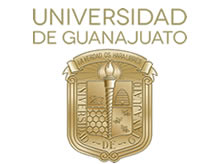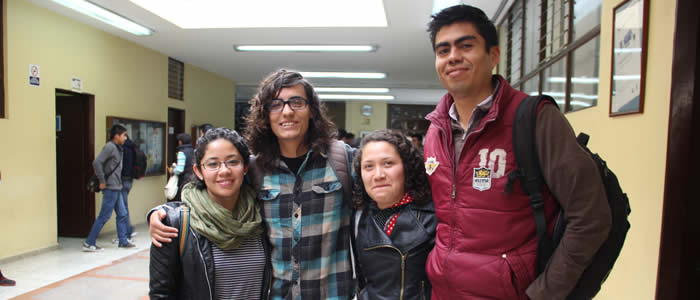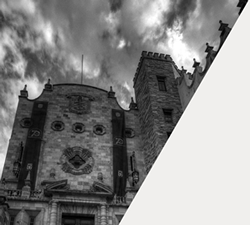Aim

Prepare professionals with a solid knowledge in mathematics as a science and method with the capability to use them in problem-solving like economy, biology and general industry.

The interested student must have an intellectual curiosity, taste for mathematics, learning desire, perseverance and discipline.

A graduate in this bachelor will be capable of reasoning rigorously and abstract. Besides planting, proving, applying, teach, extend, expose, correlate and create concepts and solve new problems. WIll be able to use new methodologies and scientific computing technologies which are an essential tool for the contemporary mathematician.

Work field

Depending on the specialization area, a graduate can work in:

• Teaching & Research
• Industry
• Software development
• Mathematic modeling in economy, biology, physics, chemistry amongst others.
Modality

Its modality is a credit system

Study Plan

The study plan includes a total of 354 credits, from which 176 are from mandatory areas (common core), 104 to optional assignments, 32 to the concentration area, 18 to human studies subjects and 24 to thesis seminar. The school periods are semiannual.

• Mandatory area, Algebra:
34 credit minimum
• Elemental Mathematics
• Superior Algebra
• Linear Algebra
• Linear Algebra
• Modern Algebra
• Algebra Select Topics
• Mandatory area, Analysis:
56 credit minimum
• Differential & Integral Calculus I
• Differential & Integral Calculus II
• Differential & Integral Calculus III
• Differential & Integral Calculus IV
• Complex Variable
• Mathematical Analysis I
• Mathematical Analysis II
• Analysis Select Topics
• Mandatory Area, Computing:
26 credit minimum
• Computing Introduction
• Computing & Algorithms
• Numeric Methods
• Computing Select Topics
• Discrete Mathematics
• Algorithms Analysis I
• Logical Analysis
• Computer Graphing
• Object-oriented Programming & Design
• Interface Design
• Logical Mathematic
• Integer Programming
• Linear Programming
• Software Engineering
• Computing Theory
• Data Base Systems
• Compilers
• Symbolic Computing
• Logical & Functional Programming
• Non-linear Programming
• Professional Practice
• Computer Architecture
• Large Databases
• Computing Tools for Science
• Cellular Neural and Automat Networks
• Non-classical Logics
• Computing Probabilistic Methods
• Computer Networks
• Image Digital Processing
• Computational linguistic
• Distributed & Parallel Processes
• Semantics & Verification
• Mandatory area, Statistics:
26 credit minimum
• Statistics & Probabilistic Elements
• Probability
• Statistic Methods
• Statistic Select Topics
• Mandatory area, Differential equations:
16 credit minimum
• Ordinary Differential Equations
• Differential Equations Select Topics
• Transformed Integrals and Special Functions
• Partial Differential Equations
• Mandatory area, Geometry:
18 credit minimum
• Geometry elements
• Modern Geometry
• Geometry Select Topics
Offered in:

Campus Guanajuato, Natural & Exact Sciences Division (DCNE)
Location Valenciana
Address: Callejón de Jalisco s/n; Mineral de Valenciana; C.P. 36240; Guanajuato, Gto.
Phone: (473) 102 61 02 & 102 61 03
Campus Webpage:www.ugto.mx/campusgto
Division Webpage:www.dcne.ugto.mxLascuráin de Retana No. 5, Col. Centro C.P. 36000

Guanajuato, Gto., México

Tel: +52 (473)  732 00 06